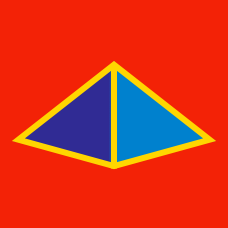Geometry

# Polyhedra: Level 4 Challenges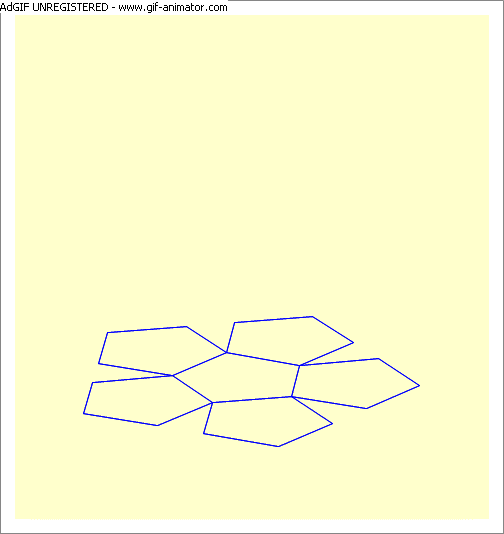A construction of a regular dodecahedron is shown in the attached animation. If the edge length is 10 units, what is the altitude of the dodecahedron ? i.e. what the distance between two opposing faces ? (Correct to two decimal places)

A point $P$ in $\mathbb{R}^{3}$ has distances of $3,7,8,9$ and $11$ from five of the vertices of a regular octahedron. If the distance from the sixth vertex of the octahedron to $P$ is $\sqrt{a}$, where $a$ is a positive, square-free integer, then find $a$.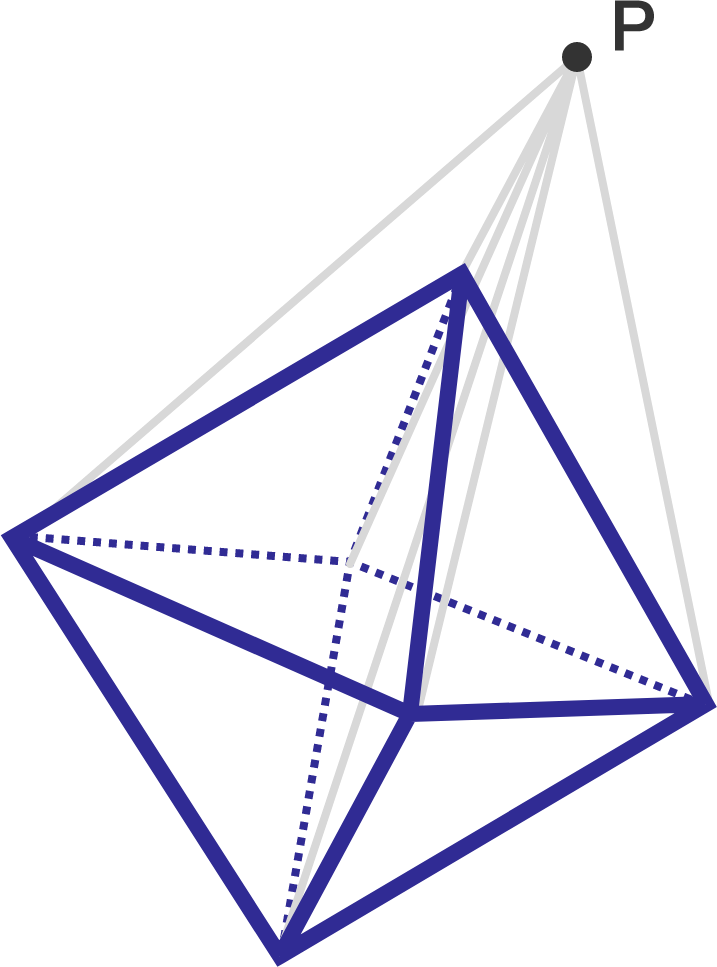This brazuca soccer ball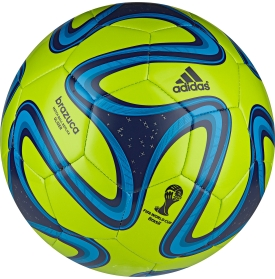has hexagonal faces, pentagonal faces, and some swirls that makes it look covered with crosses, all symmetrically arranged. It seems possible to rotate the ball so that the design realigns with itself (if we disregard printing and logos). The $5$ regular polyhedra are:

I Tetrahedron
II Cube
III Octahedron
IV Dodecahedron
V Icosahedron

A symmetry transformation is a function (or operation) $F(x)$ that leaves $x$ unchanged, that is, $F(x)=x$. If we apply, say, the same symmetry transformation which leaves leaves the tetrahedron unchanged to this soccerball, it will either leave the soccerball unchanged, or not. Which of these solids one can apply all the rotation symmetry transforms to both the regular polyhedron and the soccerball, and leave both unchanged?

Note that only rotation transforms will be considered here, that is, as the soccerball is in play, how can it re-align with itself? Both the seams and the swirls are to re-align with itself.

The artistic carpenter forms a tetradecahedron from a cube with side length 3". For each of the cube's vertices, he measures an inch out on the edges, and cuts out the resulting right tetrahedron from each corner, leaving 6 octagonal faces, and 8 triangular faces. The volume is $\frac{a}{b}$ where $a$ and $b$ are relatively prime positive integers and the surface area is $c+d\sqrt{e}$, and $e$ is prime. What is the sum $a+b+c+d+e$?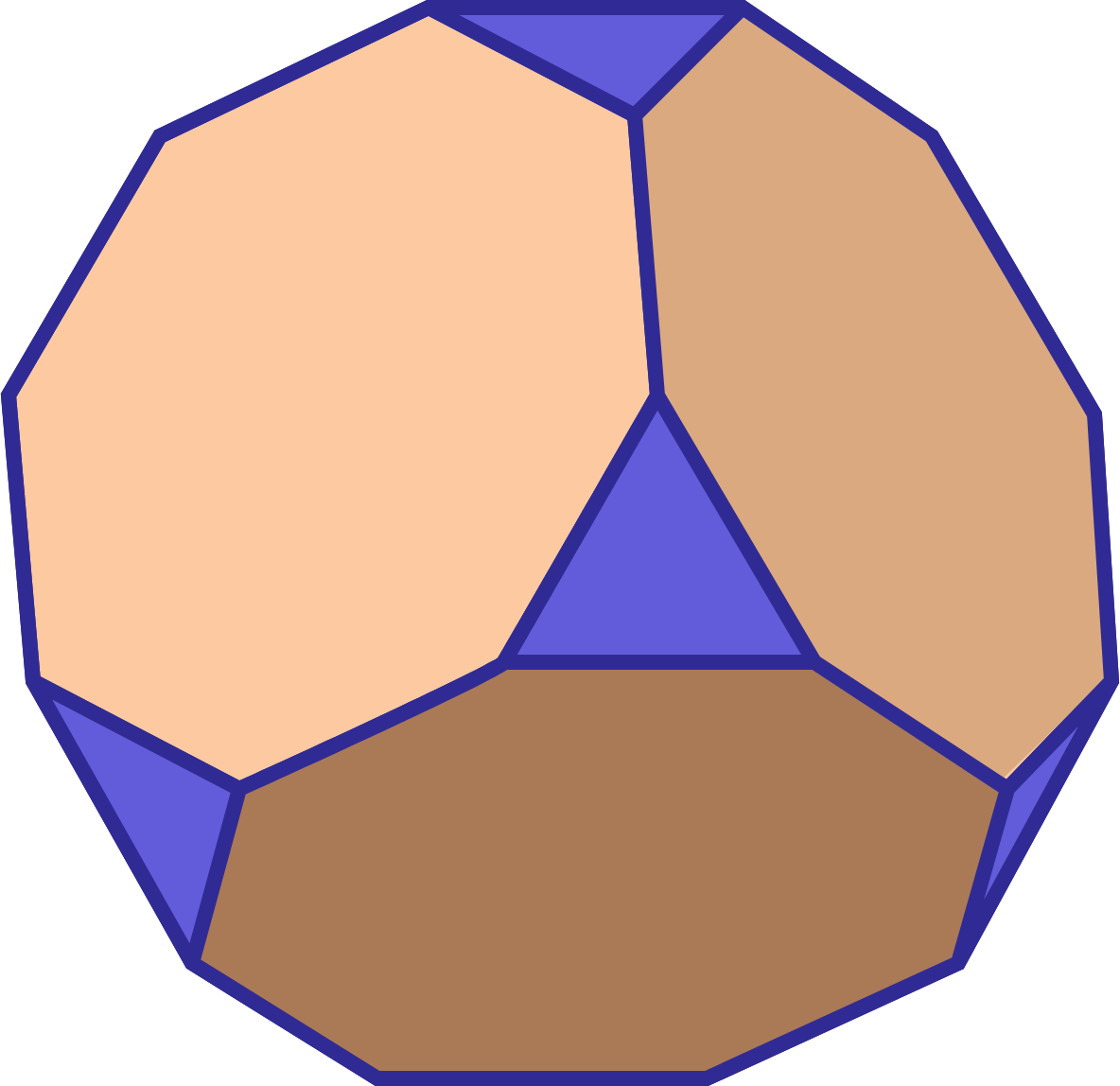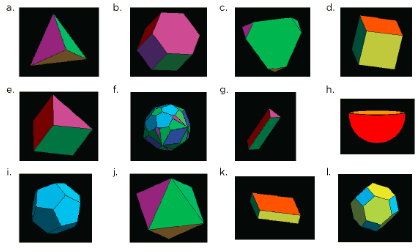If you took a solid 4-dimensional Hypercube and cut it through with a single, flat, 3D hyperplane, what might the newly-created 3D cross section look like?

×

Problem Loading...

Note Loading...

Set Loading...